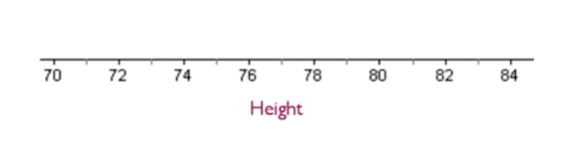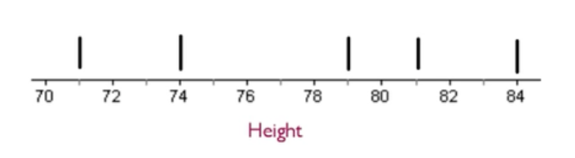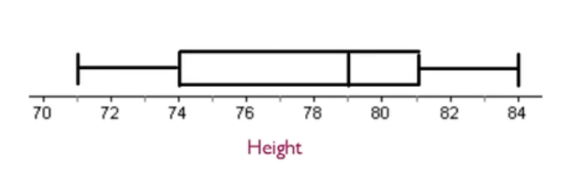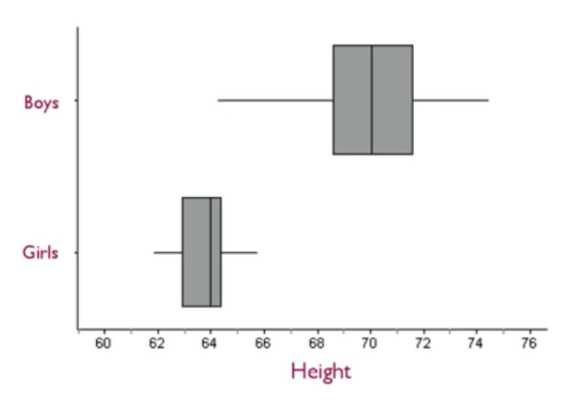+
Five Number Summary and Boxplots

# Five Number Summary and Boxplots

Author: Sophia Tutorial
##### Description:

Identify the quartiles in a box-and-whisker plot.

(more)

Sophia’s self-paced online courses are a great way to save time and money as you earn credits eligible for transfer to many different colleges and universities.*

No credit card required

37 Sophia partners guarantee credit transfer.

299 Institutions have accepted or given pre-approval for credit transfer.

* The American Council on Education's College Credit Recommendation Service (ACE Credit®) has evaluated and recommended college credit for 33 of Sophia’s online courses. Many different colleges and universities consider ACE CREDIT recommendations in determining the applicability to their course and degree programs.

Tutorial
what's covered
This tutorial will discuss the five number summary of a data set and explain box-and-whisker plots. Our discussion breaks down as follows:

1. Five Number Summary
2. Obtaining the Five Numbers
3. Box-and-Whisker Plots/Boxplots
4. Using Boxplots: Comparing Two or More Distributions

## 1. Five Number Summary

The five number summary takes larger data sets and makes them more manageable and easier to understand. By breaking down large data sets from many numbers to just five, this method can help to summarize the center and variability.

The five number summary consists of five parts:

• Minimum
• Q1
• Median
• Q3
• Maximum
term to know
Five Number Summary
A brief overview of a data set consisting of the minimum, the first quartile, the median, the third quartile, and the maximum.

## 2. Obtaining the Five Numbers

Two of the numbers in the five number summary are the smallest and largest--the minimum and the maximum.

EXAMPLE

Suppose you have a list of the heights of the Chicago Bulls basketball team:

Height of Chicago Bulls Players
Omer Asik 84
Carlos Boozer 81
Ronnie Brewer 79
Jimmy Butler 79
Luol Deng 81
Taj Gibson 81
Richard Hamilton 79
Mike James 74
Kyle Korver 79
John Lucas III 71
Joakim Noah 83
Derrick Rose 75
Brian Scalabrine 81
Marquis Teague 74
C.J. Watson 74

It's easy to see that the shortest person on the team is 71 inches tall, and the tallest person on the team is 84 inches tall. Those are two of the numbers in the five number summary. The three remaining numbers will be based on the median.

The median measures the center of a data set; it's the middle of an ordered set of data. Currently, this is alphabetical by the last name, so it needs to be rearranged from least to greatest height order. We can then see that the middle number, 79, is the median.

 71 74 74 74 75 79 79 79 79 81 81 81 81 83 84 Median

Dividing at that point, you are left with two groups: a low group and a high group. Next, take the median of each of those data sets. Now you have 74 in the low group, 81 in the high group, and 79 in the middle.

 71 74 74 74 75 79 79 79 79 81 81 81 81 83 84 Q1 MedianQ2 Q3

In this data set, 74 is the first quartile, 79 is the second quartile or the median, and 81 is the third quartile.

Now, the five number summary consists of the following five numbers.

• Minimum
• First quartile (Q1)
• Second Quartile/Median (Q2)
• Third quartile (Q3)
• Maximum.

 71 74 74 74 75 79 79 79 79 81 81 81 81 83 84 Minimum ~25% Q1 ~25% Median ~25% Q3 ~25% Maximum

The benefits of this particular summary are that about 25% of the data falls within each of these bands.

You'll notice that:

• 25% of the data falls at or below the first quartile
• 50% falls at or below the median
• 75% falls at or below the third quartile
• All the data falls at or below the maximum

Also, you can see where a concentration of data values lie within the data set. For instance, there are more data values in a narrower range. There are the same amount of data values between 79 and 81, as there are between 74 and 79. Although it's the same number of data values, the range of the 79 to 81 band is narrower than the 74 to 79 band. Therefore, you can tell the data are more clustered together in the 79 to 81 band versus the 74 to 79 band.

terms to know

Quartiles
The values that divide the data set into four equal partitions.
First/Lower Quartile
The number at which approximately 25% of the data set falls at or below that value.
Second Quartile/Middle Quartile/Median
The number at which approximately 50% of the data set falls at or below that value.
Third/Upper Quartile
The number at which approximately 75% of the data set falls at or below that value.

## 3. Box-and-Whisker Plots/Boxplots

Boxplots are also sometimes called box-and-whisker plots. A boxplot is a way to graphically display the five number summary for a data set. It is composed of a box, which contains the middle 50% of the values, and whiskers, which extend out to the maximum and minimum values.

To create a boxplot, following the simple steps below:

step by step

Step 1: Draw an axis. It can be horizontal or vertical.
Step 2: Scale the axis with equal increments.
Step 3: Make a mark to identify the five numbers from the five number summary.
Step 4: Draw a box from the first quartile to the third quartile. Draw a whisker from Q1 to the minimum and from Q3 to the maximum.

EXAMPLE

Refer to the chart above of the heights of the Chicago Bulls basketball team. Recall that the five number summary consists of:

• Minimum: 71
• Q1: 74
• Median: 79
• Q3: 81
• Maximum: 84

So, how do you put this information into a boxplot?

step by step

Step 1: Draw an axis. It can be horizontal or vertical.
Step 2: Scale the axis with equal increments. Here, the graph includes the lowest number, 71, to the tallest number, 84.Step 3: Make a mark to identify the five numbers from the five number summary: 71, 74, 79, 81, and 84.Step 4: Draw a box from the first quartile to the third quartile. The box shows where the middle 50% of the data lies. Then, about 25% percent of the data falls in the "whisker" to the left side, and about 25% of the data falls in a "whisker" to the right side. This is why it's sometimes called a box-and-whisker plot.term to know

Boxplot/Box-and-Whisker Plot
A graphical distribution of the five number summary. The "box" in the middle contains the middle 50% of the values, and the "whiskers" extend out to the maximum and minimum values from the quartiles.

## 4. Using Boxplots: Comparing Two or More Distributions

You can use boxplots to compare two distributions. For instance, if you were talking about the heights of girls versus boys, you might be able to compare them by saying the spread, or the variation, with the girls, is much less than the variation with boys.You can see this variation not only in the width of the boxes but also in the total width from the minimum to the maximum in each of these two data sets. Therefore, you can use boxplots as sort of a summary distribution for the boys and the girls.

summary
The five number summary is a brief overview of a data set consisting of the minimum, the first quartile, the median, the third quartile, and the maximum. It allows us to understand where clusters of data points might be and where the data might be more spread out. Boxplots allow you to display, visually, the five number summary. You can interpret a boxplot to see where the data points are close together and where the data points are further apart. With boxplots, you can analyze for data skews or look for symmetry. You can use multiple boxplots on the same set of axes to compare two or more distributions.

Good luck!

Source: Adapted from Sophia tutorial by Jonathan Osters.

Terms to Know
Boxplot/Box-and-Whisker Plot

A graphical distribution of the five number summary .The "box" in the middle contains the middle 50% of the values, and the "whiskers" extend out to the maximum and minimum values from the quartiles.

First/Lower Quartile

The number at which approximately 25% of the data set falls at or below that value.

Five Number Summary

A brief overview of a data set consisting of the minimum, the first quartile, the median, the third quartile, and the maximum.

Quartiles

The values that divide the data set into four equal partitions.

Second Quartile/Middle Quartile/Median

The number at which approximately 50% of the data set falls at or below that value.

Third/Upper Quartile

The number at which approximately 75% of the data set falls at or below that value.

Rating